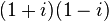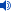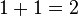# 2 (number) facts for kids

Kids Encyclopedia Facts
(Redirected from Two)
Pronunciation of the number 2
 ← 1 2 3 →
Cardinal two
Ordinal 2nd (second / twoth)
Numeral system binary
Factorization prime
Gaussian integer factorization$(1 + i)(1 - i)$
Prime 1st
Divisors 1, 2
Roman numeral II
Roman numeral (unicode) Ⅱ, ⅱ
Greek prefix di-
Latin prefix duo- bi-
Old English prefix twi-
Binary 102
Ternary 23
Quaternary 24
Quinary 25
Senary 26
Octal 28
Duodecimal 212
Vigesimal 220
Base 36 236
Greek numeral β'
Arabic ٢
Urdu
Ge'ez
Bengali
Chinese numeral 二，弍，贰，貳
Devanāgarī
Telugu
Tamil
Hebrew ב (Bet)
Khmer
Korean 이，둘
Thai

2 (Two;i/ˈt/) is a number, numeral, and glyph. It is the number after 1 and the number before 3. In Roman numerals, it is II.

## In mathematics

Two has many meanings in math. For example:$1 + 1 = 2$. An integer is even if half of it equals an integer. If the last digit of a number is even, then the number is even. This means that if you multiply 2 times anything, it will end in 0, 2, 4, 6, or 8.

Two is the smallest, first, and only even prime number. The next prime number is three. Two and three are the only prime numbers next to each other. The even numbers above two are not prime because they are divisible by 2.

Fractions with 2 in the bottom do not yield infinite.

Two is the framework of the binary system used in computers. The binary way is the simplest system of numbers in which natural numbers (0-9) can be written.

Two also has the unique property that 2+2 = 2·2 = 22 and 2! + 2 = 22.

Powers of two are important to computer science.

The square root of two was the first known irrational number.2 (number) Facts for Kids. Kiddle Encyclopedia.